# Adding a horizontal Average Line to a Chart or Graph

This post explains how to add a horizontal average line to an existing Excel chart or graph in excel. How do I add a horizontal average line to a chart or graph gives loads of context for the viewer. How to use Excel VBA Macro to add a horizontal average line to the existing chart in Excel.

For example, you have a simple Excel spreadsheet tracking product sales. And you have a column with dates and another with sales. And you have made a simple chart with both columns as data sources. And you want to add a horizontal average line to this chart so that the user can see how close they are to their average sales. You can follow this guide to achieve the result.

## Adding a horizontal Average Line to a Chart or Graph

To add a horizontal average line to a chart, just do the following steps:

#1 create a new average line column that contains average sales data. And type the following formula into the first cell of average column. =AVERAGE(B2:D2), then drag the AutoFill Handle over to the average columns.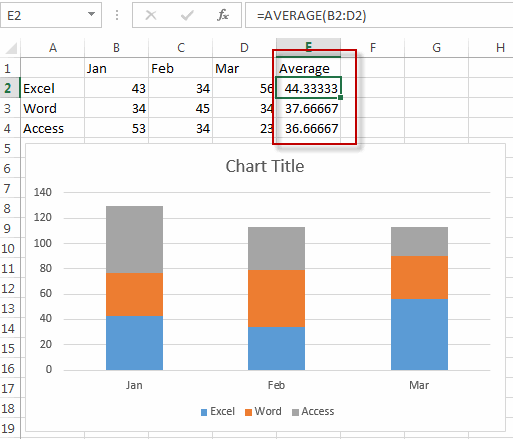#2 Right-click on the existing chart, and choose Select Data… from the drop-down menu list.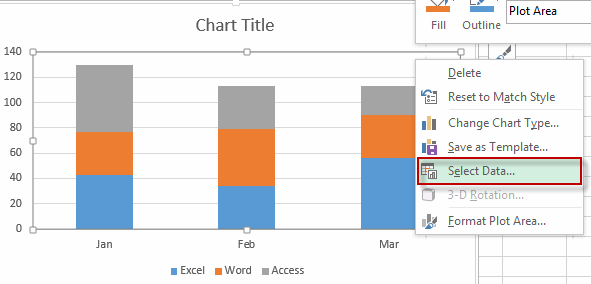#3 the Select Data source window will appear.

#4 click Add button under Legend Entries section, the Edit Series window will appear.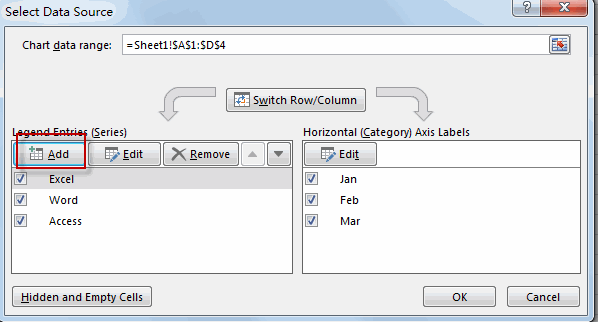#5 enter one Series name such as: Average line into the Series name text box. Then select average line column as its series values. Then click OK button.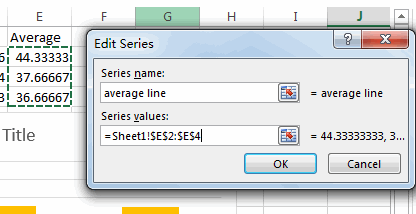#6 you will see that the average line has been added into the cart.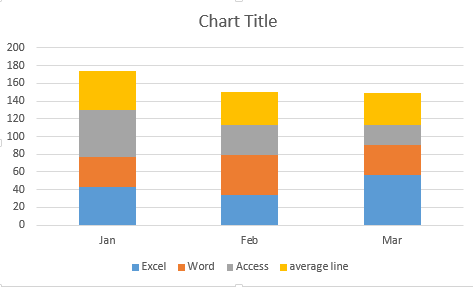#7 Right-click on the newly average line series, and select Change Series Chart Type from the drop down menu list. The Change Chart Type window will appear.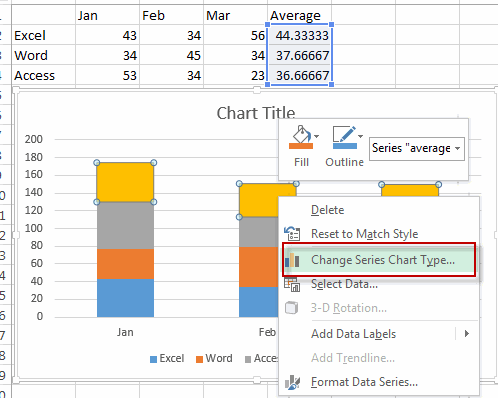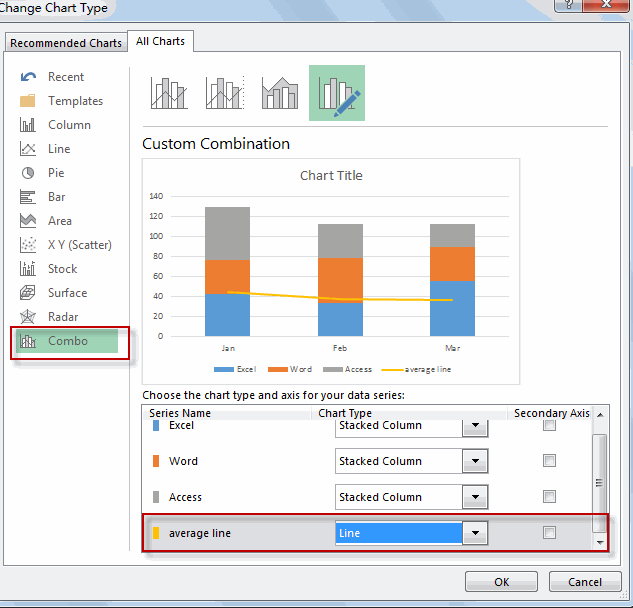#8 Select chart type as line for the average line series.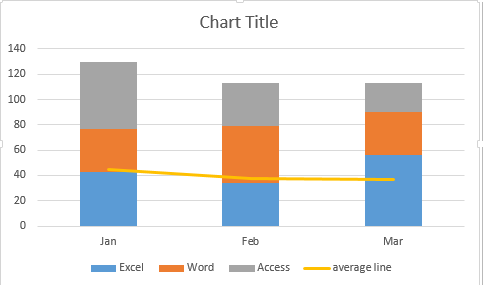So far, the horizontal average line is added into the existing chart.

## Adding a horizontal Average Line to a Chart or Graph with VBA

You can also write an Excel VBA Macro to add a horizontal average line to an excel chart, just do the following steps:

#1 click on “Visual Basic” command under DEVELOPER Tab.#2 then the “Visual Basic Editor” window will appear.

#3 click “Insert” ->”Module” to create a new module.#4 paste the below VBA code into the code window. Then clicking “Save” button.```Sub AddAverageLineChart()
Dim S As Series
Dim A As Variant
Dim T As Double
Dim O As Variant
If VBA.TypeName(Application.Selection) <> "Series" Then Exit Sub
Set S = Application.Selection
A = S.Values
T = Application.WorksheetFunction.Average(A)
ReDim O(LBound(A) To UBound(A))
For i = LBound(O) To UBound(O)
O(i) = T
Next
With ActiveChart.SeriesCollection.NewSeries
.XValues = S.XValues
.Values = O
.Name = "Average line" & S.Name
.AxisGroup = S.AxisGroup
.MarkerStyle = xlNone
.Border.Color = S.Border.Color
.ChartType = xlLine
.Format.Line.ForeColor.ObjectThemeColor = msoThemeColorAccent6
End With
End Sub```

#5 select all data of columns in your chart.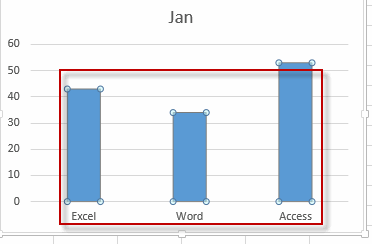#6 back to the current worksheet, then run the above excel macro. Click Run button.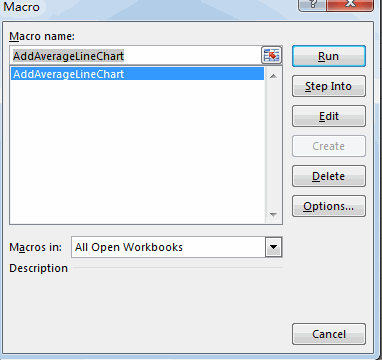#7 Let’s see the result.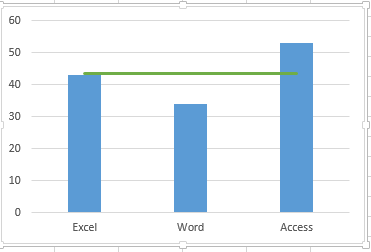### Related Functions

• Excel AVERAGE function
The Excel AVERAGE function returns the average of the numbers that you provided.The syntax of the AVERAGE function is as below:=AVERAGE (number1,[number2],…)….